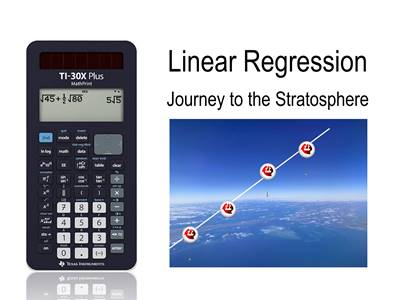# Activities

•• ##### AuthorAust Senior

50 Minutes

## Linear Regression Journey#### Activity Overview

In this activity model a linear relationship by fitting an appropriate line of best fit to a scatterplot and using it to describe and quantify associations related to time and altitude for a weather balloon. Students make predictions based on the model and check them using the associated video.

This activity includes a video tutorial.

#### Objectives

• Fit a Least Squares Regression line
• Interpret the intercept and gradient
• Extrapolate and Interpolate
• Understand Least Squares method

#### Vocabulary

• Least Squares Regression
• Independent variable
• Dependent variable
• Mean (average)
• Extrapolate
• Interpolate